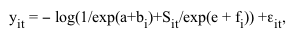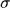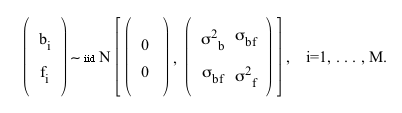### Nicholas J. Barrowman, Ransom A. Myers, Ray Hilborn, Daniel G. Kehler, and Chris A. Field. 2003. The variability among populations of Coho salmon in the maximum reproductive rate and depensation. Ecological Applications 13:784–793.

Appendix A. A description of models and methods for combining population dynamics data.

In Eq. 2, let logi = a + bi and log(Rmax,i) = e + fi, which leads towhere yit = log[ Rit / Sit ]. Several different models are possible here:

#### Model 0 (Individual data sets model)

Treat a, b1,..., b M, e, f1, ..., fM as fixed.

This is the standard model used in population ecology. It is based on the assumption that there is no relationship among populations of a taxonomic group.

#### Model I (Random's, fixed Rmax's model)

Treat a, e, f1, ..., fM as fixed; assume b1, ...,bM ~ iid N(0,2b) .

This model, featuring population-specific fixed effects, was used in the linear case, i.e., the Ricker spawner-recruitment model, by Myers et al. (1999). This model works well in the linear case, but often not in the nonlinear case unless the estimates of spawner abundance cover a wide range for most populations. Model I may lead to estimation difficulties because of the large number of fixed effects. In simulations we have found that Model I leads to overestimates of a and underestimates of2b, i.e., the mean of logis overestimated and its variance underestimated (Kehler 2001). Also, since each additional population introduces an additional parameter, the asymptotic properties of maximum likelihood-type estimates for this model may not be good (Jiang 1996). For example, depending on the conditions assumed, the estimates may not be consistent or asymptotically normal, making it difficult to obtain valid inferences.

#### Model II (Random's and Rmax's model)

Treat a and e as fixed and assumeIn this model, all parameters are random. In our models, we will constrain the correlation ofand asymptotic recruitment to be zero,bf = 0, because we have no reason to assume that the among-population variability in these parameters should be correlated. This will be the basic model that we apply to data.

#### Approaches to inference for hierarchical models

Nonlinear mixed effects models belong to the general class of hierarchical models. Recently, the hierarchical Bayes approach has enjoyed considerable popularity, because it permits estimation of a very broad class of models and may produce more complete assessments of the uncertainty of parameter estimates. However it requires specification of prior distributions for the fixed effects and variance components, which may be challenging. Furthermore, estimation, typically using Markov Chain Monte-Carlo (MCMC) methods, can be time consuming and determination of convergence may be difficult.

A traditional mixed model approach is an alternative, and software currently exists for both linear and nonlinear models of this type if Gaussian observational errors and random effects are assumed. Here we concentrate on this approach because of its relative simplicity and reliability. We obtain estimates for our models using the approximate maximum likelihood algorithm of Lindstrom and Bates (1990). The output from our analyses could serve as starting values and checks in a hierarchical Bayes approach.

In addition to providing overall estimates of ecological parameters and among-population variances, hierarchical models can also provide improved population-specific estimates. The unknown values of the random effects that represent population-specific values of ecological parameters are termed "realized" random effects. Estimates of these realized random effects have traditionally been called "predictions" and their Bayesian analogues are known as predictive distributions. (Such terminology is slightly misleading, however, since the estimates do not necessarily pertain to future events.)

The Lindstrom and Bates approach uses empirical Bayes estimates of realized random effects. The reason for this terminology comes from considering the distributions of the random effects as prior distributions. If the parameters of the priors (i.e., the variances and covariances) were known, then standard Bayesian calculations would give posterior distributions for the random effects, and point estimates could be obtained using, for example, posterior means. Since the parameters of the priors are unknown, the empirical Bayes approach is to substitute estimates of the parameters of the priors and then proceed as if using true priors (e.g., Myers et al. 1999).

In contrast, the hierarchical Bayes approach puts priors on the parameters of the random effects distributions. We sometimes refer to the hierarchical Bayes approach as "fully Bayes" to distinguish it from the empirical Bayes approach and hybrid approaches involving specification of prior distributions for some but not all parameters (e.g., Liermann and Hilborn 1997). If the hyperpriors are uninformative and the marginal likelihood of the hyperparameters is relatively peaked, the empirical Bayes and fully Bayes approaches should give similar results (Smith 1983).

Literature cited

Jiang, J. 1996. REML estimation: asymptotic behavior and related topics. Ann. Statist. 24(1):255–286.

Kehler, D. G. 2001. Bias in linear and nonlinear mixed effects models. Master's thesis, Dalhousie University, Halifax, Nova Scotia, Canada.

Liermann, M., and Hilborn, R. 1997. Depensation in fish stocks: A hierarchic Bayesian meta-analysis. Canadian Journal of Fisheries and Aquatic Sciences 54:1976–1985.

Lindstrom, M. J., and Bates, D. M. 1990. Nonlinear mixed effects models for repeated measures data. Biometrics 46:673–687.

Myers, R. A., Bowen, K. G., and Barrowman, N. J. 1999. The maximum reproductive rate of fish at low population sizes. Canadian Journal of Fisheries and Aquatic Sciences 56:2404–2419.

Smith, A. F. M. 1983. Comment on: "Bayes methods for combining the results of cancer studies in humans and other species"[J. Amer. Statist. Assoc. 78 (1983), no. 382, 293-308] by William H. DuMouchel and Jeffrey E. Harris. J. Amer. Statist. Assoc. 78(382):310–311.

[Back to A013-012]